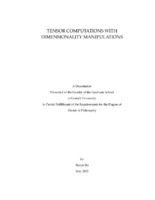## Tensor computations with dimensionality manipulations##### Abstract
Methodologies that ensure the compressibility of tensors are introduced. Bounds on the storage costs with respect to various tensor formats are derived. A new algorithm combining data-sparse tensor formats and factored alternating direction implicit method is designed to solve Sylvester tensor equations, and incorporated in a fast spectral Poisson equation solver on cubes with optimal complexity. New parallelizable algorithms for computing the tensor-train decomposition of tensors in original format, streaming data, Tucker format, and that satisfy algebraic relations, are proposed. Based on the input format, the algorithms involve deterministic or probabilistic aspects, and all have guarantees of accuracy. Scaling analysis and numerical experiments are provided to demonstrate computational and storage efficiency. An ultraspherical spectral method is developed for fractional partial differential equations via the Caffarelli--Silvestre extension on disk and rectangular domains. A parallel domain decomposition solver is designed for multi-core performance of non-smooth functions. The discretized equation is solved via direct tensor equation solvers, and numerical performance is shown with a fractional PDE constrained optimization problem. Linear systems in electron correlation calculation from computational chemistry are converted into tensor equations to reduce computing and storage costs. Several algorithms are developed to exploit the sparsity and data-sparsity of chemical structures. Numerical results indicate that tensor equation solvers are competitive over traditional linear system solvers with both canonical and localized orbital bases formulations. The quantized tensor-train format of tensors is introduced to approximate analytic functions via Chebyshev polynomial expansions. Analysis of different types of singularities is carried out, leading to theoretical guarantees of coefficient storage compressibility.
200 pages
2022-05
##### Keywords
Approximation theory; Numerical analysis; Numerical linear algebra; Scientific computing
##### Committee Chair
Townsend, Alex John